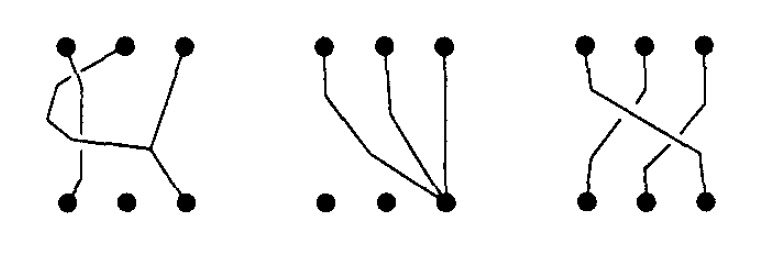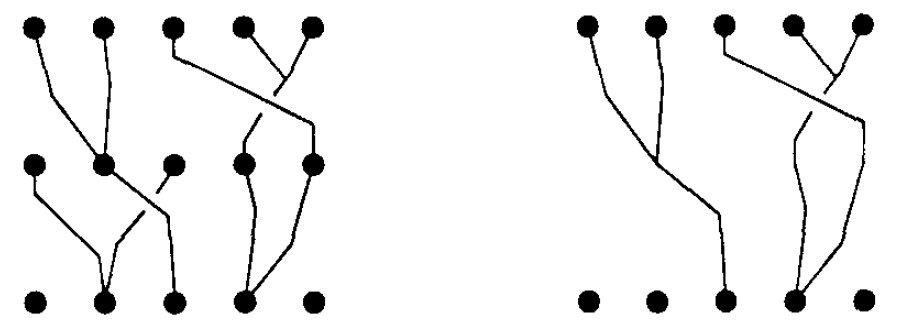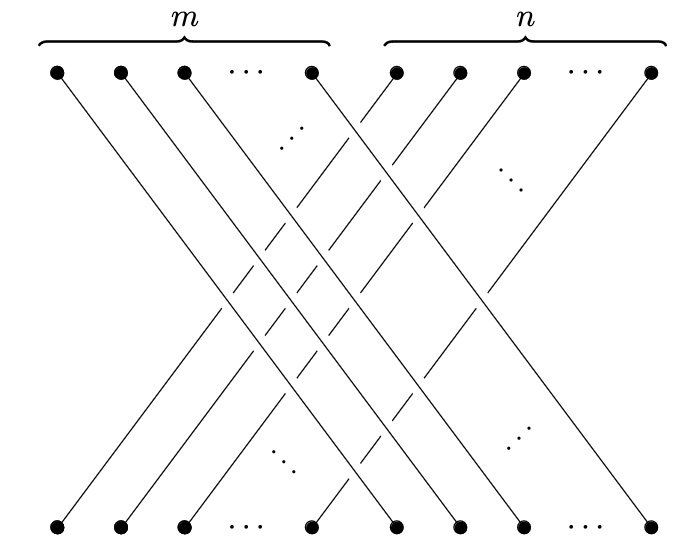# nLab vine

Contents

### Context

#### Monoidal categories

monoidal categories

With braiding

With duals for objects

With duals for morphisms

With traces

Closed structure

Special sorts of products

Semisimplicity

Morphisms

Internal monoids

Examples

Theorems

In higher category theory

# Contents

## Idea

Lavers (1997) introduced the monoid $V_n$ of $n$-vines, whose elements are thought of as paths between two sets of $n$ distinct points in $\mathbb{R}^3$ which are allowed to merge into a single path, but not separate again. Some examples, from Lavers’ original paper, are depicted below.Notice that $V_n$ is not a group because a general vine cannot be untangled to give the trivial vine which is $n$ paths straight down. However, via pre- and post-composition, the vine monoid is acted on by the braid group on $n$ strands. When two $n$-vines are composed any resulting strands which are only attached to one endpoint get retracted down to that endpoint, as in the following image (again from Lavers 97):More generally, one can consider vines from $n$ points to $m$ points. As a result vines can be assembled into a category, as described below.

## Definition

What is called the category of vines is the free braided strict monoidal category containing a braided monoid (i.e. an $E_2$-algebra). It is also the PROB for braided monoids.

Concretely:

###### Definition

Let

• $P_I \coloneqq \big\{ (i,0,1) \,\big\vert\, i=1, 2, \ldots, m \big\}$

• $P_T \coloneqq \big\{ (i,0,0) \,\big\vert\, i=1, 2, \ldots,n \big\}$

be linearly ordered collections of points in $\mathbb{R}^3$.

Then an $(m,n)$-vine is a set of arcs $\{v_1,\ldots,v_m\}$ (i.e. piecewise linear maps from $[0,1]$ to $\mathbb{R}^3$) with the following properties:

1. $v_i(0)=(i,0,1)$,

2. $v_i(1)\in P_T$,

3. for all $h\in [0,1]$, $v_i(h)$ has $z$-coordinate $1-h$,

4. if $v_i(t)=v_j(t)$ for any $t\in (0,1]$ then $v_i(s)=v_j(s)$ for all $t\leq s\leq 1$.

###### Definition

Let $\mathbb{V}$ be the category with set of objects the natural numbers with set of morphisms $\mathbb{V}(m,n)$ being $(m,n)$-vines modulo a suitable notion of ambient isotopy that allows arcs to be deformed up to homotopy but not pass through one another.

## Properties

The monoidal structure of $\mathbb{V}$ is given by addition of natural numbers and juxtaposition of vines. Because $\mathbb{V}$ contains the braid category as a subcategory, it cannot be symmetric monoidal, but it is braided monoidal. The braiding is the same as that of the braid category, the “$m$-over-$n$” braid depicted below.###### Exercise

Show that the above is a braided monoidal structure on $\mathbb{V}$.

• T. G. Lavers, The theory of vines, Comm. Algebra 25 4 (1997) 1257–1284 [doi:10.1080/00927879708825919]

• Mark Weber, §6.3 of: Internal algebra classifiers as codescent objects of crossed internal categories. Theory and Applications of Categories, 30 50 (2015) 1713–1792 [tac:30/50, pdf]